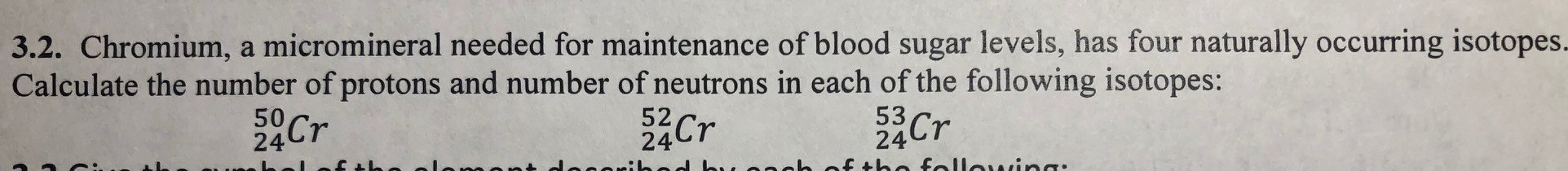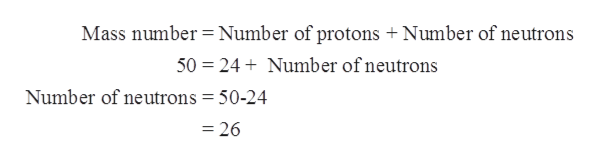3.2. Chromium, a micromineral needed for maintenance of blood sugar levels, has four naturally occurring isotopes.Calculate the number of protons and number of neutrons in each of the following isotopes:5324af tha fallawina:Cr50245224CrCrenb

Questionhelp_outlineImage Transcriptionclose3.2. Chromium, a micromineral needed for maintenance of blood sugar levels, has four naturally occurring isotopes. Calculate the number of protons and number of neutrons in each of the following isotopes: 53 24 af tha fallawina: Cr 50 24 52 24 Cr Cr enb fullscreen
Step 1

The isotopes of chromium are given as follows:

Step 2

Isotopes are the compounds having same atomic number but different mass number. To write an isotope symbol atomic number(Z) is written on the lower left side and atomic mass(A) is written on the upper left side of an element.

Step 3

Atomic number is equal to the number of protons in an element.

Mass number = Number of protons + Number of neutrons

The atomic and mass number for first isotope of chromium is 24 and 50 respectively.

Since, atomic number = numbe...help_outlineImage TranscriptioncloseMass number Number of protons + Number of neutrons 50 24Number ofneutrons Number of neutrons = 50-24 =26 fullscreen

Want to see the full answer?

See Solution

Want to see this answer and more?

Our solutions are written by experts, many with advanced degrees, and available 24/7

See Solution
Tagged in

General Chemistry